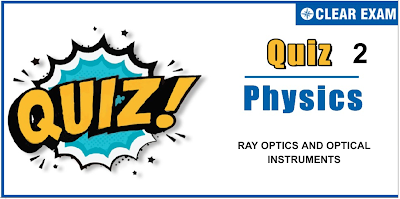## ATOMS QUIZ-2

JEE Advanced Physics Syllabus can be referred by the IIT aspirants to get a detailed list of all topics that are important in cracking the entrance examination. JEE Advanced syllabus for Physics has been designed in such a way that it offers very practical and application-based learning to further make it easier for students to understand every concept or topic by correlating it with day-to-day experiences. In comparison to the other two subjects, the syllabus of JEE Advanced for physics is developed in such a way so as to test the deep understanding and application of concepts.

Q1. A photon collides with a stationary hydrogen atom in ground state inelastically. Energy of the colliding photon is 10.2 eV. After a time interval of the order of micro second another photon collides with same hydrogen atom inelastically with an energy of 15n eV. What will be observed by the detector?
•  2 photon of energy 10.2 eV.
•  2 photon of energy of 1.4 eV.
•  One photon of energy 10.2 eV and an electron of energy 1.4 eV
•  One photon of energy 10.2 eV and another photon of energy 1.4 eV
Solution
(c) The first photon will excite the hydrogen atom (in ground state) in first excited state (as E_2-E_1-10.2 eV). Hence, during de-excitation a photon of 10.2 eV will be released. The second photon of energy 15 eV can ionize the atom. Hence the balance energy ie, (15-13.6) eV = 1.4 eV is retained by the electron. Therefore, by the second photon an electron of energy 1.4 eV will be released.
Q2. A hydrogen-like atom emits radiations of frequency 2.7×10^15 Hz when it makes a transition from n=2 to n=1. The frequency emitted in a transition from n=3 to n=1 will be
•   1.8×10^15 Hz
•  3.2×10^15 Hz
•  4.7×10^5 Hz
•  6.9×10^15 Hz
Q3. Imagine an atom made of a proton and a hypothetical particle of double the mass of the electron but having the same charge as the electron. Apply the Bohr atom model and consider all possible transitions of this hypothetical particle to the first excited level. The longest wavelength photon that will be emitted has wavelength [given in terms of the Rydberg constant R for the hydrogen atom] equal to
•   9/5R
•  36/5R
•  18/5R
•  4/R

Q4. In a hydrogen atom, the electron is in n^th excited state. It comes down to first excited state by emitting 10 different wavelengths. The value of n is
•  6
•  7
•  8
•  9
Solution
(a) Number of possible emission lines are n(n-1)/2 when an electron jumps from n^th state to ground state. In this question, this value should be (n-1)(n-2)/2 Hence, 10=((n-1)(n-2))/2 Solving this, we get n=6
Q5. If the series limit wavelength of the Lyman series for hydrogen atom is 912 Å, then the series limit wavelength for the Balmer series for the hydrogen atom is
•  912 Å/2
•  912 Å
•  912×2 Å
•  912×4 Å
Q6. An electron in the ground state of hydrogen has an angular momentum L1 and an electron in the first excited state of lithium has an angular momentum L2. Then
•  L1=L2
•  L1=4L2
•  L2=2L1
•  L1=2L2
Q7. According to Bohr’s theory of hydrogen atom, the product of the binding energy of the electron in the nth orbit and its radius in the nth orbit
•  Is proportional to n2
•  Is inversely proportional to n3
•  Has a constant value of 10.2 eV-Å
•  Has a constant value of 7.2 eV-Å
Q8. The electron in a hydrogen atom makes a transition n1→n2, where n1 and n2 are the principal quantum numbers of the two states. Assume Bohr model is valid in this case. The frequency of the orbital motion of the electron in the initial state is 1/27 of that in the final state. The possible values of n1 and n2 are
•  n1=6,n2=3
•  n1=4,n2=2
•  n1=8,n2=1
•  n1=3,n2=1
Q9. Mark out the correct statement regarding X-rays
•  When fast moving electrons strike the metal target, they enter the metal target and in a very short time span come to rest, and thus an accelerated charged electron produces electromagnetic waves (X-rays)
•  Characteristic X-rays are produced due to transition of an electron from higher energy levels to vacant lower energy levels
•  rays spectrum is a discrete spectra just like hydrogen spectra
•  Both (a) and (b) are correct
Solution
(b) Option (a) explains the production of X-rays on the basis of electromagnetic theory of light, which is not able to explain the characteristic X-rays and cut-off wavelength, so option (a) is wrong Option (b) correctly explains the production of characteristic X-rays Option (c) is wrong as X-ray spectra is a continuous spectra having some peaks representing characteristic X-rays

Q10. The potential difference across the Coolidge tube is 20 kV and 10 mA current flows through the voltage supply. Only 0.5% of the energy carried by the electrons striking the target is converted into X-rays. The power carried by the X-ray beam is P. Then
•  P=0.1 W
•  P=1 W
•  P=2 W
• P=10 W#### Written by: AUTHORNAME

AUTHORDESCRIPTION## Want to know more

Please fill in the details below:

## Latest NEET Articles\$type=three\$c=3\$author=hide\$comment=hide\$rm=hide\$date=hide\$snippet=hide

Name

ltr
item
BEST NEET COACHING CENTER | BEST IIT JEE COACHING INSTITUTE | BEST NEET, IIT JEE COACHING INSTITUTE: Atoms - Quiz 2
Atoms - Quiz 2
https://1.bp.blogspot.com/-Dsc9SRg-3ic/YNw9ZVY-fUI/AAAAAAAAPLM/Y1DQforzkmMdLuoQrDiDgq_tLg06PAIVQCLcBGAsYHQ/s400/2.png
https://1.bp.blogspot.com/-Dsc9SRg-3ic/YNw9ZVY-fUI/AAAAAAAAPLM/Y1DQforzkmMdLuoQrDiDgq_tLg06PAIVQCLcBGAsYHQ/s72-c/2.png
BEST NEET COACHING CENTER | BEST IIT JEE COACHING INSTITUTE | BEST NEET, IIT JEE COACHING INSTITUTE
https://www.cleariitmedical.com/2021/07/atoms-quiz-2.html
https://www.cleariitmedical.com/
https://www.cleariitmedical.com/
https://www.cleariitmedical.com/2021/07/atoms-quiz-2.html
true
7783647550433378923
UTF-8